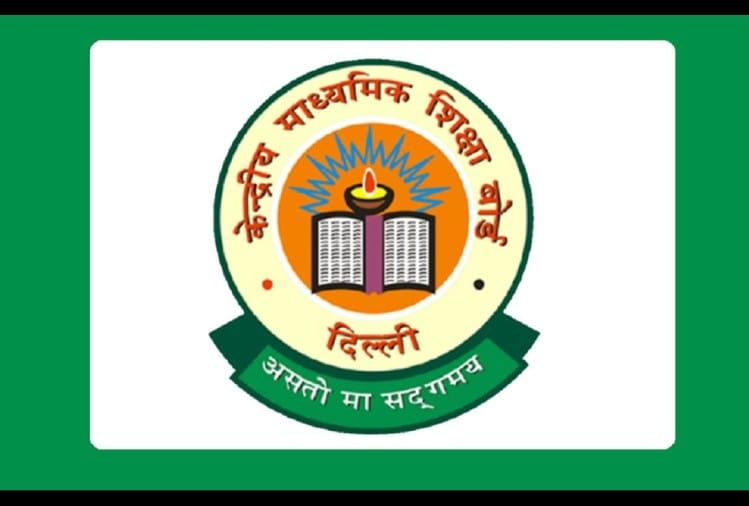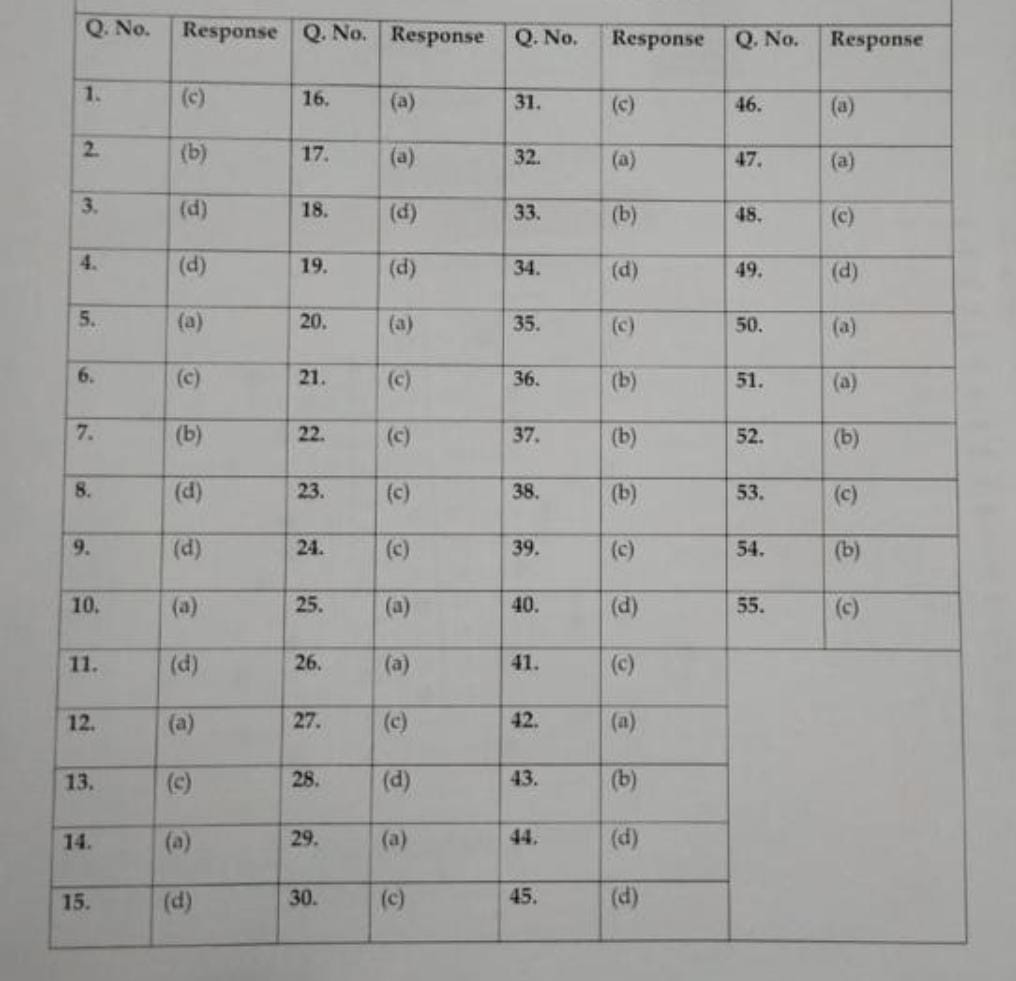CBSE Class 12 Term 1 Board 2021-22 - PC : My Result Plus

CBSE Class 12th Physics subject Term-1 exam was held on Friday, 10 December. The examination was conducted from 11.30 am to 01.00 pm. The total time of the paper for 35 marks was 90 minutes. Many students found some questions very difficult, while there were some questions in which the students got confused. There were some students who found the paper balanced. Overall, there was a mixed response from the students. At the same time, teachers say that the paper came from the syllabus taught to the students.

A total of 55 multiple choice questions were asked by CBSE in class 12th Physics paper. Out of which the students had to answer 45 questions. There were a total of three sections - A, B and C in this paper. A total of 25 questions were asked in Section-A out of which any 20 questions were to be answered. Whereas Section-B had 24 questions and out of these, any 20 questions were to be answered. Six questions were asked in Section-C, out of which the students were required to answer five questions. At the same time, solving each question will score 0.7 marks.

A total of 51% questions were numerical based and 10 questions were very difficult Talking to the Amar Ujala Digital Education team, Manoj Sharma, a physics subject teacher of Rajkiya Pratibha Vikas Vidyalaya (RPVV), Dwarka Sector-10, Delhi, said that out of 55 questions, a total of 28 questions were numerical based. That is, 51 percent of the questions which came were numerical. The difficulty level of 10 questions out of 55 questions was very high.

CBSE teacher Manoj Sharma says that the Physics paper of 35 marks was challenging for average students. Average students can get 16 to 17 marks in this paper. At the same time, good performing students can get 32 to 34 marks out of 35. Average students would have found it difficult to solve 25 to 30 questions. On the other hand, students performing well must have attempted 40 to 45 questions. Many students have found this paper to be too long as compared to the duration of 90 minutes. The teachers said that the paper came from NCERT books.Analysis of Section B

Many students said that a lot of numerical questions were asked in Section-B. Due to which calculation had to be done to solve each question and it was time consuming. In this sense, the Physics paper was lengthy. The exam was balanced for those students who had practiced a lot from the sample paper of Physics subject.